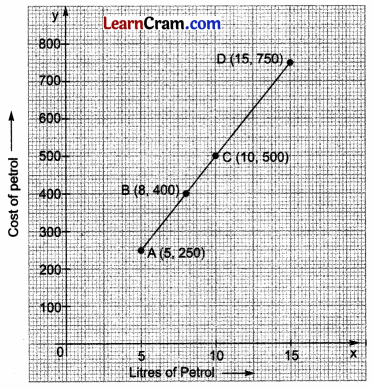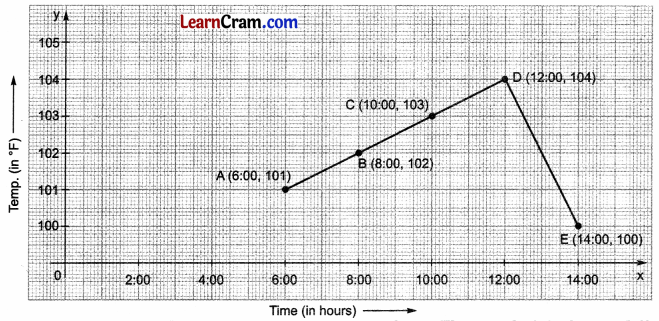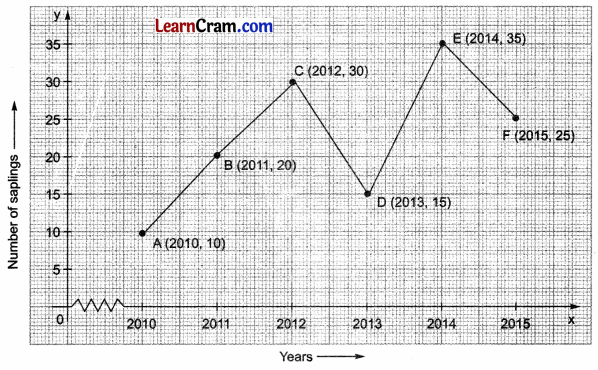# DAV Class 8 Maths Chapter 13 Worksheet 2 Solutions

The DAV Maths Book Class 8 Solutions Pdf and DAV Class 8 Maths Chapter 13 Worksheet 2 Solutions of Introduction to Graphs offer comprehensive answers to textbook questions.

## DAV Class 8 Maths Ch 13 WS 2 Solutions

Question 1.
The following table gives the temperature at 12:00 noon on seven successive days in Delhi.

 Day (October) 1 2 3 4 5 6 7 Temp. (in °C) 14 18 16 13 19 20 17

Plot a graph to show this information.
Solution:
Scale on x-axis: 1 day = 1 cm
Scale on y-axis: 5°C = 1 cmQuestion 2.
The quantity of petrol filled in a bike and the cost of petrol are given in the following table:

 Litres of Petrol Filled 5 8 10 15 Cost of Petrol 250 400 500 750

Draw a graph to show this information.
Solution:
Scale on the x-axis: 5 units = 2 cm
Scale on the y-axis: 100 units = 1 cmQuestion 3.
The temperature of a patient, admitted to the hospital with viral fever, at different times of the day is given below. Draw the temperature-time graph to represent the data:

 Time (in hours) 6:00 8:00 10:00 12:00 14:00 Temp. (in °F) 101 102 103 104 100

Solution:
Scale on x-axis: 1 hr = 1 cm
Scale on y-axis: 1° = 1 cmQuestion 4.
A bus is going on a long journey starting at 4:00 hour. The speed of the bus at different hours is given below:

 Time (in hours) 4:00 6:00 8:00 10:00 12:00 14:00 Speed (in km/hr) 30 50 60 45 80 70

Draw a speed-time graph for the above data.
Solution:
Scale on the x-axis: 1 hr = 1 cm
Scale on the y-axis: 10 km/hr = 1 cmQuestion 5.
The following table shows the amount of wheat grown by Heera in different years:

 Years 1995 1996 1997 1998 1999 2000 Wheat grown (in quintals) 240 220 160 250 270 300

Plot a graph to illustrate this information.
Solution:
Scale on x-axis: 1 year = 2 cm
Scale on y-axis: 100 quintal = 2 cm### DAV Class 8 Maths Chapter 13 Value Based Questions

Question 1.
Given below is the number of saplings planted by the Eco-Club members of a school in different years.

 Year 2010 2011 2012 2013 2014 2015 Number of saplings 10 20 30 15 35 25

(i) Plot a graph for this information.
(ii) What other activities can be taken by Eco-Club members? Name anyone.
(iii) What value of the Eco-Club members is depicted here?
Solution:
(i) Scale on x-axis, 1 unit = 1 year
Scale on the y-axis, 1 unit = 5 saplings(ii) Say no to plastic bags, reduce the use of electricity, and take care of animals, birds, plants, etc.
(iii) Eco-Club members take care of the environment.